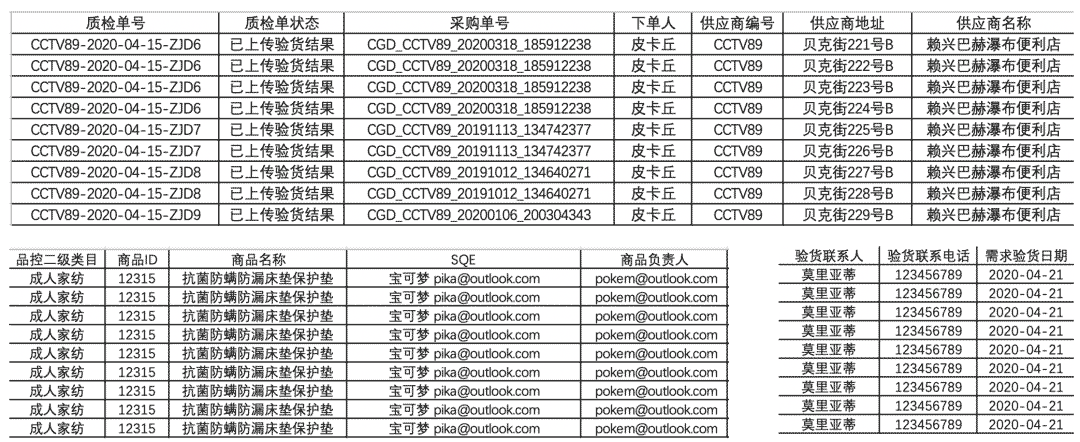﻿ Python办公自动化从Excel中计算整理数据并写入Word_python_脚本之家
python# Python办公自动化从Excel中计算整理数据并写入Word

## 前言

• openpyxl读取Excel获取内容
• docx读写Word文件

## 需求确认OK，需求分析完毕，接下来看Python如何解决！

## Python实现

```from openpyxl import load_workbook
import os
# 获取桌面的路径
def GetDesktopPath():
return os.path.join(os.path.expanduser("~"), 'Desktop')
path = GetDesktopPath() + '/资料/' # 形成文件夹的路径便后续重复使用
sheet = workbook.active # 获取当前页
# 可以用代码获取数据范围，如果要批处理循环迭代也方便
# 获取有数据范围
print(sheet.dimensions)
# A1:W10```

```cells = sheet['A1:A4']  # 返回A1-A4的4个单元格
cells = sheet['A'] # 获取A列
cells = sheet['A:C'] # 获取A-C列
cells = sheet # 获取第5行
# 注意如果是上述用cells获取返回的是嵌套元祖
for cell in cells:
print(cell.value) # 遍历cells依然需要取出元祖中元素才可以获取值
# 获取一个范围的所有cell
# 也可以用iter_col返回列
for row in sheet.iter_rows(min_row=1, max_row=3,min_col=2, max_col=4):
for cell in row:
print(cell.value)
```

```# SQE
SQE = sheet['Q2'].value
# 供应商&制造商
supplier = sheet['G2'].value
# 采购单号
C2_10 = sheet['C2:C10'] # 返回cell.tuple对象
# 利用列表推导式后面同理
vC2_10 = [str(cell.value) for cell in C2_10]
# 用set简易去重后用,连接，填word表用
order_num = ','.join(set(vC2_10))
# 用set简易去重后用&连接，word文件名命名使用
order_num_title = '&'.join(set(vC2_10))
# 产品型号
T2_10 = sheet['T2:T10']
vT2_10 = [str(cell.value) for cell in T2_10]
ptype = ','.join(set(vT2_10))
# 产品描述
P2_10 = sheet['P2:P10']
vP2_10 = [str(cell.value) for cell in P2_10]
info = ','.join(set(vP2_10))
info_title = '&'.join(set(vP2_10))
# 日期
# 用datetime库获取今日时间以及相应格式化
import datetime
today = datetime.datetime.today()
time = today.strftime('%Y年%m月%d日')
# 验货数量
V2_10 = sheet['V2:V10']
vV2_10 = [int(cell.value) for cell in V2_10]
total_num = sum(vV2_10) # 计算总数量
# 验货箱数
W2_10 = sheet['W2:W10']
vW2_10 = [int(cell.value) for cell in W2_10]
box_num = sum(vW2_10)
# 生成最终需要的word文件名
title = f'{order_num_title}-{supplier}-{total_num}-{info_title}-{time}-验货报告'
print(title)```

```# pip install pypiwin32
from win32com import client
docx_path = path + '模板.docx'
# doc转docx的函数
def doc2docx(doc_path,docx_path):
word = client.Dispatch("Word.Application")
doc = word.Documents.Open(doc_path)
doc.SaveAs(docx_path, 16)
doc.Close()
word.Quit()
print('\n doc文件已转换为docx \n')
if not os.path.exists(docx_path):
doc2docx(docx_path[:-1], docx_path)```

```docx_path = path + '模板.docx'
from docx import Document
# 实例化
document = Document(docx_path)
# 读取word中的所有表格
tables = document.tables
# print(len(tables))
# 15```

```tables.cell(1, 1).text = SQE
tables.cell(1, 1).text = supplier
tables.cell(2, 1).text = supplier
tables.cell(3, 1).text = ptype
tables.cell(4, 1).text = info
tables.cell(5, 1).text = order_num
tables.cell(7, 1).text = time``````for i in range(2, 11):
tables.cell(i, 0).text = str(sheet[f'T{i}'].value)
tables.cell(i, 1).text = str(sheet[f'P{i}'].value)
tables.cell(i, 2).text = str(sheet[f'C{i}'].value)
tables.cell(i, 4).text = str(sheet[f'V{i}'].value)
tables.cell(i, 5).text = str(sheet[f'V{i}'].value)
tables.cell(i, 6).text = '0'
tables.cell(i, 7).text = str(sheet[f'W{i}'].value)
tables.cell(i, 8).text = '0'
tables.cell(12, 4).text = str(total_num)
tables.cell(12, 5).text = str(total_num)
tables.cell(12, 7).text = str(box_num)```• word写入的数据需是字符串，所以从Excel获取的数据需要用str格式化
• 表格可能存在合并等其他情况，因此你看到的行数和列数可能不是真实的，需要用代码不断测试。

```document.save(path + f'{title}.docx')
print('\n文件已生成')```